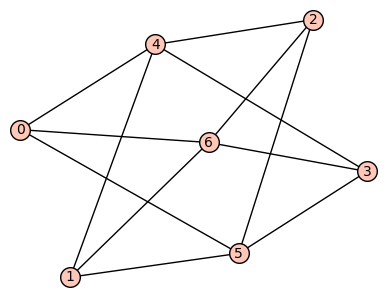# Graphs having highest second smallest laplacian eigen value from a collectionAnonymous

Using this code

for G in graphs(7):
if G.girth()==4:
L = G.laplacian_matrix().eigenvalues()
L.sort()
show(L)
G.show()


I have generated all graphs on $7$ vertices having girth=4. Now, from this code can we get the only unique graph having largest algebraic connectivity among all others.

edit retag close merge delete

Sort by » oldest newest most votedFor convenience we can define

algebraic_connectivity = lambda g: sorted(g.laplacian_matrix().eigenvalues())


Then we can obtain at once

G = max((g for g in graphs(7) if g.girth() == 4), key=algebraic_connectivity)


But this assumes there is only one maximum. If we want to be more on the safe side:

my_graphs = [g for g in graphs(7) if g.girth() == 4]
from collections import defaultdict
acs = defaultdict(list)
for g in my_graphs:
ac = QQbar(algebraic_connectivity(g)) # ensure keys are always in QQbar, even if rational
acs[ac].append(g)
max_ac = max(acs.keys())
print('There are {} graphs of (maximal) algebraic connectivity {}'.format(len(acs[max_ac]), max_ac))
for g in acs[max_ac]:
show(g)


Output:

There are 1 graphs of (maximal) algebraic connectivity 3more

Ok. But suppose we use this code for all connected graphs on $8$ vertices and choose girth=$5$. Then this code is not giving the correct result

Why do you say it's not correct? When I run G = max((g for g in graphs(8) if g.is_connected() and g.girth() == 5), key=algebraic_connectivity) (or more efficiently G = max((g for g in graphs.nauty_geng('8 -c') if g.girth() == 5), key=algebraic_connectivity)) (or the longer code with analogous modification) then it seems correct.

@Kuldeep you're right, I got the definition of algebraic connectivity wrong because Wikipedia was not clear. I updated both Wikipedia and my answer.

No problem. But this time your answer is perfect. Very good. Thank you

You're welcome. You can vote and accept using the buttons to the left of the answer.